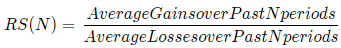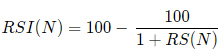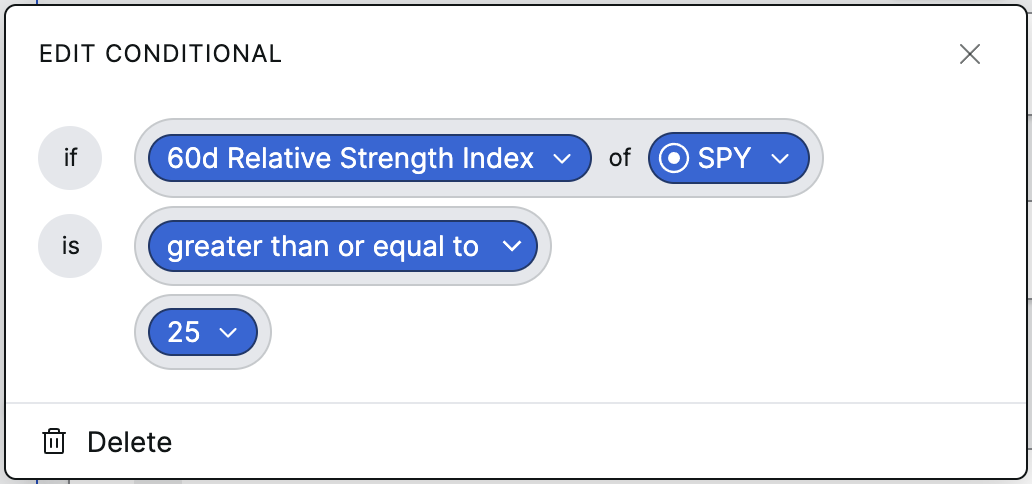# Relative strength index

What is RSI?

RSI is a technical indicator used to measure momentum in a certain look-back period, signaling whether the asset is overbought/oversold. The RSI will be an oscillator between 0 and 100, attempting to measure the momentum of price. Values above 70 signaling an asset may be overbought (and therefore overvalued), while 30 and below signals the asset is oversold (and therefore undervalued), in the time period selected.

Formula

There are two steps to calculating RSI, shown below:

The first step is to calculate the relative strength over a look-back period of N days. Say we’re looking at a period of two weeks—for average gains, we add together every day that closed in a gain, with days that ended in losses equaling 0, and divide it by N. The same is done for average losses, only vice versa—we add together the return of every day that ended in a loss, with days that ended in gains equaling 0, and divide it by N. We then divide the average gains by the average losses.The second step is normalizing the relative strength and turning it into an indicator that oscillates between 0 and 100. We take the output from step one, add 1 to it, then divide 100 by that number. From there, we subtract it from 100 to find our RSI in the specified look-back period.This creates an index that roughly measures the momentum of the asset in relation to the average gains or losses taken on the asset in the specified time period.

We use up to 1000-days of smoothing, in order to minimize a loss function.

Where do you see RSI in Composer?RSI can be used in conditional and filter blocks. In the example above, we’d be taking the 60-day RSI of SPY and comparing it to the value 25. The conditional will be "true" if the 60-day RSI of SPY is greater than 25. A value below 25 would signal that SPY is oversold, and may be under-valued as a result.

RSI can also be used in filter blocks—for example, grouping 10 assets together and selecting the bottom 2 or 3 RSI values, in order to hold assets that are considered under-valued.